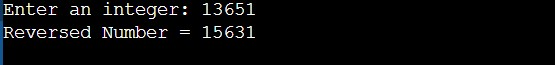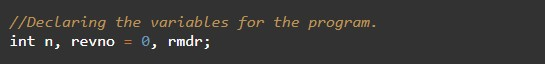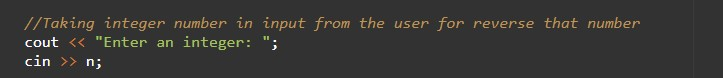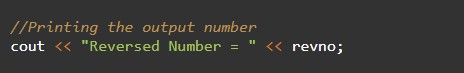# C++ Programs to Reverse any Number

In this tutorial you will learn about the C++ Programs to Reverse any Number and its application with practical example.

## C++ Programs to Reverse any Number

In this tutorial, we will learn to create a C++ program that will Reverse any Number using C++ programming.

## Prerequisites

Before starting with this tutorial we assume that you are best aware of the following C++ programming topics:

• Operators in C++ Programming.
• Basic Input and Output function in C++ Programming.
• Basic C++ programming.
• For loop in C++ programming.
• Conditional Statements in C++ programming.
• Arithmetic operations in C++ Programming.

## Program to Reverse any Number:-

In c++ programming, it is possible to take integer input from the user and Reverse any Number with the help of a C++ program. The reversing of a number means the digits of a number will be swapped from the first position to the last position. The C++ language has many types of header libraries that have supported functions in them with the help of these files the programming is easy.

With the help of this program, we can Reverse any Number.

## Output:-In the above program, we have first initialized the required variable.• num= it will hold the integer value of the input.
• n = it will hold the integer value.
• digit = it will hold the integer value.
• rev = it will hold the integer value.

Input message for the user for the integer value.Program Logic Code.Printing output Reverse any Number.In this tutorial we have learn about the C++ Programs to Reverse any Number and its application with practical example. I hope you will like this tutorial.